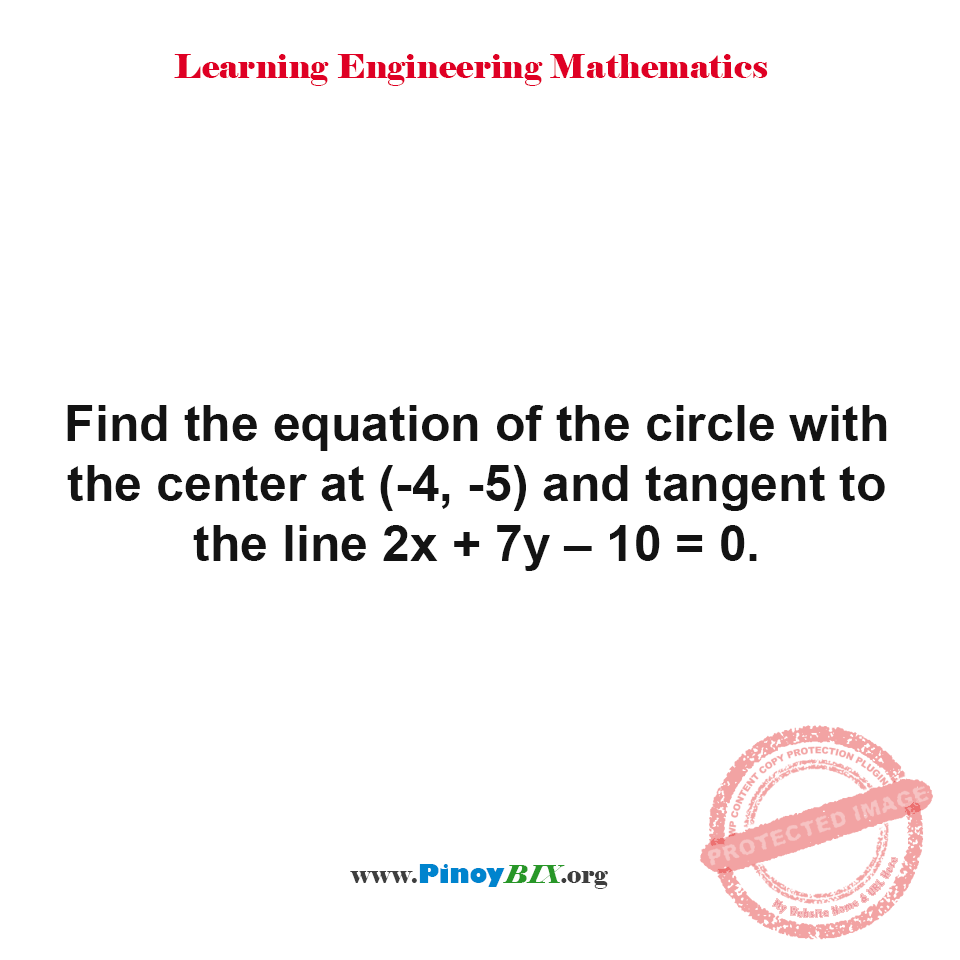# Solution: Find the equation of the circle given the center and tangent to the line

(Last Updated On: January 19, 2020)#### Problem Statement:

Find the equation of the circle with the center at (-4, -5) and tangent to the line 2x + 7y – 10 = 0.

The equation of the circle is x^2 + y^2 + 8x + 10y – 12 = 0.

#### Latest Problem Solving in Analytic Geometry Problems (Circles, Parabola, Ellipse, Hyperbola)

More Questions in: Analytic Geometry Problems (Circles, Parabola, Ellipse, Hyperbola)

#### Online Questions and Answers in Analytic Geometry Problems (Circles, Parabola, Ellipse, Hyperbola)

PinoyBIX Engineering. © 2014-2021 All Rights Reserved | How to Donate? |